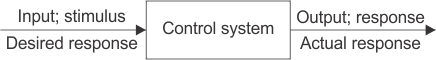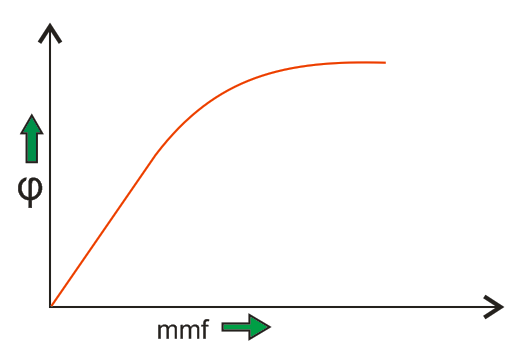Control system engineering is the branch of engineering which deals with the principles of control theory to design a system which gives ...

Control system engineering is the branch of engineering which deals with the principles of control theory to design a system which gives desired behavior in a controlled manner. Hence, this is interdisciplinary. Control system engineers analyze, design, and optimize complex systems which consist of highly integrated coordination of mechanical, electrical, chemical, metallurgical, electronic or pneumatic elements. Thus control engineering deals with diverse range of dynamic systems which include human and technological interfacing.Control system engineering focuses on analysis and design of systems to improve the speed of response, accuracy and stability of system. The two methods of control system include classical methods and modern methods. The mathematical model of system is set up as first step followed by analysis, designing and testing. Necessary conditions for the stability are checked and finally optimization follows.

In classical method, mathematical modeling is usually done in time domain, frequency domain or complex s domain. Step response of a system is mathematically modeled in time domain differential analysis to find its settling time, % overshoot etc. Laplace transforms are most commonly used in frequency domain to find the open loop gain, phase margin, band width etc of system. Concept of transfer function, sampling of data, poles and zeros, system delays all comes under the classical control engineering stream.

Modern control engineering deals with Multiple Input Multiple Output (MIMO) systems, State space approach, Eigen values and vectors etc. Instead of transforming complex ordinary differential equations, modern approach converts higher order equations to first order differential equations and solved by vector method.

Automatic control systems are most commonly used as it does not involve manual control. The controlled variable is measured and compared with a specified value to obtain the desired result. As a result of automated systems for control purposes, the cost of energy or power as well as the cost of process will be reduced increasing its quality and productivity.

## Historical Review of Control Engineering

The application of Automatic control system is believed to be in use even from the ancient civilizations. Several types of water clock were designed and implemented to measure the time accurately from the third century BC, by Greeks and Arabs. But the first automatic system is considered as the Watts Fly ball Governor in 1788, which started the industrial revolution. The mathematical modeling of Governor is analyzed by Maxwell in 1868. In 19th century, Leonhard Euler, Pierre Simon Laplace and Joseph Fourier developed different methods for mathematical modeling. The second system is considered as Al Butz’s Damper Flapper - thermostat in 1885. He started the company now named as Honeywell.

The beginning of 20th century is known as the golden age of control engineering. During this time classical control methods were developed at the Bell Laboratory by Hendrik Wade Bode and Harry Nyquist. Automatic controllers for steering ships were developed by Minorsky, Russian American Mathematician. He also introduced the concept of Integral and Derivative Control in 1920s. Meanwhile the concept of stability was put forward by Nyquist and followed by Evans. The transforms were applied in control system by Oliver Heaviside. Modern Control Methods were developed after 1950s by Rudolf Kalman, to overcome the limitation of classical Methods. PLC’s were introduced in 1975.

Before I introduce you the theory of control system it is very essential to know the various types of control systems. Now there are various types of systems, we are going to discuss only those types of systems that will help us to understand the theory of control system and detail description of these types of system are given below:

### Linear Control Systems

In order to understand the linear control system, we should know the principle of superposition. The principle of superposition theorem includes two the important properties and they are explained below:

Homogeneity : A system is said to be homogeneous, if we multiply input with some constant ‘A’ then output will also be multiplied by the same value of constant (i.e. A).

Additivity: Suppose we have a system ‘S’ and we are giving the input to this system as ‘a1’ for the first time and we are getting output as ‘b1’ corresponding to input ‘a1’. On second time we are giving input ‘a2’ and correspond to this we are getting output as ‘b2’. Now suppose this time we giving input as summation of the previous inputs ( i.e. a1 + a2 ) and corresponding to this input suppose we are getting output as (b1 + b2) then we can say that system ‘S’ is following the property of additivity. Now we are able to define the linear control systems as those types of control systems which follow the principle of homogeneity and additivity.

#### Examples of Linear Control System

Consider a purely resistive network with a constant DC source. This circuit follows the principle of homogeneity and additivity. All the undesired effects are neglected and assuming ideal behavior of each element in the network, we say that we will get linear voltage and current characteristic. This is the example of linear control system.

### Non-linear Systems

We can simply define non linear control system as all those system which do not follow the principle of homogeneity. In practical life all the systems are non-linear system.

#### Examples of Non-linear System

A well known example of non-linear system is magnetization curve or no load curve of a DC machine. We will discuss briefly no load curve of DC machines here: No load curve gives us the relationship between the air gap flux and the field winding mmf. It is very clear from the curve given below that in the beginning there is a linear relationship between winding mmf and the air gap flux but after this, saturation has come which shows the non linear behavior of the curve or characteristics of the non linear control system.### Analog or Continuous System

In these types of control system we have continuous signal as the input to the system. These signals are the continuous function of time. We may have various sources of continuous input signal like sinusoidal type signal input source, square type of signal input source, signal may be in the form of continuous triangle etc.

### Digital or Discrete System

In these types of control system we have discrete signal (or signal may be in the form of pulse) as the input to the system. These signals have the discrete interval of time. We can convert various sources of continuous input signal like sinusoidal type signal input source, square type of signal input source etc into discrete form using the switch.

Now there are various advantages of discrete or digital system over the analog system and these advantages are written below:

1. Digital systems can handle non linear control systems more effectively than the analog type of systems.
2. Power requirement in case of discrete or digital system is less as compared to analog systems.
3. Digital system has higher rate of accuracy and can perform various complex computations easily as compared to analog systems.
4. Reliability of digital system is more as compared to analog system.
5. Digital system works on the logical operations which increases their accuracy many times.
6. Losses in case of discrete systems are less as compared to analog systems in general.

### Single Input Single Output Systems

These are also known as SISO type of system. In this the system has single input for single output. Various example of this kind of system may include temperature control, position control system etc.

### Multiple Input Multiple Output Systems

These are also known as MIMO type of system. In this the system has multiple outputs for multiple inputs. Various example of this kind of system may include PLC type system etc.

### umped Parameter System

In these types of control systems the various active (resistor) and passive parameters (like inductor and capacitor) are assumed to be concentrated at a point and that’s why these are called lumped parameter type of system. Analysis of such type of system is very easy which includes differential equations.

### Distributed Parameter System

In these types of control systems the various active (resistor) and passive parameters (like inductor and capacitor) are assumed to be distributed uniformly along the length and that’s why these are called distributed parameter type of system. Analysis of such type of system is slightly difficult which includes partial differential equations.Name

BASIC ELECTRICAL,12,BATTERIES,4,CIRCUIT THEORIES,9,CONTROL SYSTEMS,3,DC MOTOR,1,DIGITAL ELECTRONICS,1,DISTRIBUTED GENERATION,2,DISTRIBUTION,6,ELECTRICAL DRIVES,1,ELECTRICAL LAWS,8,ELECTRONICS DEVICES,2,General,7,GENERATION,3,GENERATOR,1,HIGH VOLTAGE,4,ILLUMINATION,1,INDUCTION MOTOR,7,MATERIALS,1,MEASUREMENT,1,MOTOR,1,POWER ELECTRONICS,2,PROJECTS ON INDUCTION MOTOR,1,PROTECTION,1,SMART GRID,3,SWITCHGEAR,4,SYNCHRONOUS MOTOR,1,TRANSFORMER,6,TRANSMISSION,4,
ltr
item
Electrical for Us: Control Engineering | Linear and Non Linear Control System
Control Engineering | Linear and Non Linear Control System